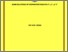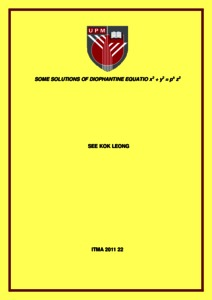# Some solutions of diophantine equation x3+y3=pk z3

## Citation

See, Kok Leong (2011) Some solutions of diophantine equation x3+y3=pk z3. Masters thesis, Universiti Putra Malaysia.

## Abstract

Diophantine equation is an algebraic equation in two or more variables in which solutions to it are from some predetermined classes and it is one of the oldest branches of number theory. They are studied and investigated to determine the values of the variables that satisfy the equation. This activity began since time immemorial and has developed into branches according to types of the equations. This research concentrates on investigating the solvability and the determination of representations of the integral solutions of the diophantine equation x3 + y3 = pk z3 where k is a positive integer, k º/ 0(mod3) and p = 2, 3, 5, 13. The approach used to determine the solutions of the equation is first to look for mathematical patterns of the integral solutions to the diophantine equation x3 + y3 = pk z3. Once the patterns are obtained on the forms of the solutions, general formulae are then obtained from which solutions can be acquired. Proofs are constructed to show the validity of the formulae. In our investigation, we determine the solutions to the equation for selected values of primes under certain conditions. We also determine the condition under which as equation do not have solutions. We also show that the number of solutions, if there exist, are either finite or infinite depending on the values of the associated parameter. Our work opens up other possibilities of future work that can be carried out based on the results obtained in this research.Preview
Text
IPM 2011 22 IR.pdfView Item